Close

# PresentationTechnical Papers Fast-Forward
:
Accelerated Complex-Step Finite Difference for Expedient Deformable Simulation
Event Type
Technical Papers Fast-Forward
Registration Categories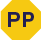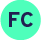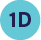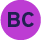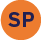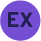TimeSunday, 17 November 201918:11 - 18:12
LocationGreat Hall 1&2
DescriptionIn deformable simulation, an important computing task is to calculate the gradient and derivative of the strain energy function in order to infer the corresponding internal force and tangent stiffness matrix. The standard numerical routine is the finite difference method, which evaluates the target function multiple times under a small real-valued perturbation. Unfortunately, the subtractive cancellation prevents us from setting this perturbation sufficiently small, and the regular finite difference is doomed for computing problems requiring a high-accuracy derivative evaluation. In this paper, we graft a new finite difference scheme, namely the complex-step finite difference (CSFD), with physics-based animation. CSFD is based on the complex Taylor series expansion, which avoids subtractions in first-order derivative approximation. As a result, one can use a very small perturbation to calculate the numerical derivative that is as accurate as its analytic counterpart. We accelerate the original CSFD method so that it is also as efficient as the analytic derivative. This is achieved by discarding high-order error terms, decoupling real and imaginary calculations, replacing costly functions based on the theory of equivalent infinitesimal, and isolating the propagation of the perturbation in composite/nesting functions. CSFD can be further augmented with multicomplex Taylor expansion and Cauchy-Riemann formula to handle higher-order derivatives and tensor-valued functions. We demonstrate the accuracy, convenience, and efficiency of this new numerical routine in the context of deformable simulation -- one can easily deploy a robust simulator for general hyperelastic materials, including user-crafted ones to cater specific needs in different applications. Higher-order derivatives of the energy can be readily computed to construct modal derivative bases for reduced real-time simulation. Inverse simulation problems can also be conveniently solved using gradient/Hessian-based optimization procedures.